# statsmodels.discrete.discrete_model.NegativeBinomial¶

class statsmodels.discrete.discrete_model.NegativeBinomial(endog, exog, loglike_method='nb2', offset=None, exposure=None, missing='none', **kwargs)[source]

Negative Binomial Model for count data

Parameters: endog : array-like 1-d endogenous response variable. The dependent variable. exog : array-like A nobs x k array where nobs is the number of observations and k is the number of regressors. An intercept is not included by default and should be added by the user. See statsmodels.tools.add_constant. loglike_method : string Log-likelihood type. ‘nb2’,’nb1’, or ‘geometric’. Fitted value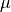Heterogeneity parameter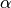nb2: Variance equal to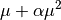(most common) nb1: Variance equal to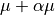geometric: Variance equal to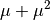offset : array_like Offset is added to the linear prediction with coefficient equal to 1. exposure : array_like Log(exposure) is added to the linear prediction with coefficient equal to 1. missing : str Available options are ‘none’, ‘drop’, and ‘raise’. If ‘none’, no nan checking is done. If ‘drop’, any observations with nans are dropped. If ‘raise’, an error is raised. Default is ‘none.’

References

References:

Greene, W. 2008. “Functional forms for the negtive binomial model
for count data”. Economics Letters. Volume 99, Number 3, pp.585-590.
Hilbe, J.M. 2011. “Negative binomial regression”. Cambridge University
Press.

Attributes

 endog (array) A reference to the endogenous response variable exog (array) A reference to the exogenous design.

Methods

 cdf(X) The cumulative distribution function of the model. cov_params_func_l1(likelihood_model, xopt, ...) Computes cov_params on a reduced parameter space corresponding to the nonzero parameters resulting from the l1 regularized fit. fit([start_params, method, maxiter, ...]) fit_regularized([start_params, method, ...]) initialize() Initialize is called by statsmodels.model.LikelihoodModel.__init__ and should contain any preprocessing that needs to be done for a model. loglike(params) Loglikelihood for negative binomial model pdf(X) The probability density (mass) function of the model. predict(params[, exog, exposure, offset, linear]) Predict response variable of a count model given exogenous variables. score_obs(params)

Attributes

 endog_names exog_names